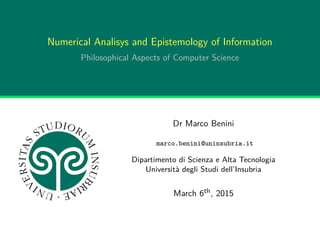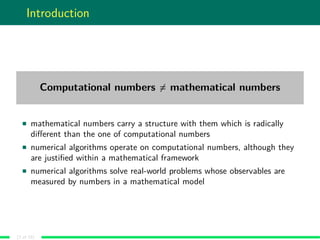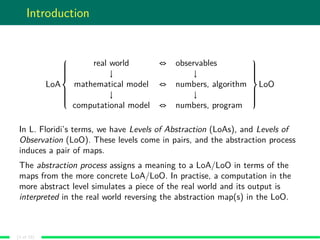Successfully reported this slideshow.

# Numerical Analysis and Epistemology of Information

1

Share×
1 of 15
1 of 15

# Numerical Analysis and Epistemology of Information

1

Share

The slides of my presentation at the workshop "Philosophical Aspects of Computer Science", European Centre for Living Technology, University “Ca’ Foscari”, Venice, March 2015.

The slides of my presentation at the workshop "Philosophical Aspects of Computer Science", European Centre for Living Technology, University “Ca’ Foscari”, Venice, March 2015.

## More Related Content

### Related Books

Free with a 14 day trial from Scribd

See all

### Related Audiobooks

Free with a 14 day trial from Scribd

See all

### Numerical Analysis and Epistemology of Information

1. 1. Numerical Analysis and Epistemology of Information Philosophical Aspects of Computer Science Dr Marco Benini marco.benini@uninsubria.it Dipartimento di Scienza e Alta Tecnologia Università degli Studi dell’Insubria March 6th, 2015
2. 2. Introduction Computational numbers = mathematical numbers mathematical numbers carry a structure with them which is radically diﬀerent than the one of computational numbers numerical algorithms operate on computational numbers, although they are justiﬁed within a mathematical framework numerical algorithms solve real-world problems whose observables are measured by numbers in a mathematical model (2 of 15)
3. 3. Introduction LoA    real world ⇔ observables ↓ ↓ mathematical model ⇔ numbers, algorithm ↓ ↓ computational model ⇔ numbers, program    LoO In L. Floridi’s terms, we have Levels of Abstraction (LoAs), and Levels of Observation (LoO). These levels come in pairs, and the abstraction process induces a pair of maps. The abstraction process assigns a meaning to a LoA/LoO in terms of the maps from the more concrete LoA/LoO. In practise, a computation in the more abstract level simulates a piece of the real world and its output is interpreted in the real world reversing the abstraction map(s) in the LoO. (3 of 15)
4. 4. Computational numbers at least two ways to interpret them: as numbers and as strings of bits ﬁnite objects to ﬁt into the computer memory in most cases bounded objects, so, e.g., there is a maximum and a minimum number (!) eﬃcient manipulation by the computer hardware within well-speciﬁed bounds, they behave as (approximations of) mathematical numbers Remark Computational numbers are formal mathematical objects, but of a diﬀerent sort which is loosely related to proper mathematical numbers. (4 of 15)
5. 5. Structure and paradoxes Example An apparent paradox: addition is not associative, even if it is f (x,y,z) = x +(y +z) g(x,y,z) = (x +y)+z In mathematics, f = g when x, y, z are numbers (N, Z, R, C). But, if computational numbers are formed by two decimal digits: f (97,4,−5) = 97+(4−5) = 97−1 = 96 g(97,4,−5) = (97+4)−5 = ??−5 = ?? Numerical stability and related phenomena are well-known to numerical analysts: these aspects must be considered in the implementation of algorithms. Moving from the mathematical LoA to the computational LoA means to abstract away part of the structure of numbers: the correctness of algorithms is preserved as far as the structure justifying them is retained. (5 of 15)
6. 6. Correctness Problem Given a pair (a,b) in R such that f (a) < 0, f (b) > 0, with f continuous and monotone, and assuming that there is a unique root R of f in the interval [a,b], calculate it. -q q qa bRr f q Newton’s method: let r = (a+b)/2 if f (r) > 0 iterate on (a,r) if f (r) < 0 iterate on (r,b) if f (r) = 0 stop It is possible to show that r approximates the root of f better and better, up to any degree of precision. Thus, Newton’s method is correct. (6 of 15)
7. 7. Correctness Consider -q q qa b R r f q Since f (r) < 0 but it is very close to zero, its representation may be 0 or even a small positive number. In both cases, the iteration of the method will fail to reﬁne the approximation of R, as |R −r| will not decrease. So Newton’s method is computationally wrong! we may think, as usual in numerical analysis, that the calculated f (r) contains an error causing the method’s failure; or, we may think that r and f (r) are points with some degree of uncertainty: when the uncertainty of f (r) is big enough, it prevents the case choice in the method, causing its failure. (7 of 15)
8. 8. Measures The relation between the real world and the mathematical LoA shows similar diﬃculties: an act of measuring is required to transform an observable into a number. And, in most cases, the uncertainty of the measure is poorly considered. Many examples from physics are immediate to show, leading to paradoxical results: the truth is that there is a long withstanding tradition in physics and engineering that analyses these problems and provide solutions. But, nevertheless, computer science uses many other mathematical models, where the problem is, at least, underestimated. (8 of 15)
9. 9. Paradoxes Example Web banking applications are very popular, nowadays. If a user has a bank account, a savings account, and some investments like bonds or stock options, the application usually shows the total capital. Strictly speaking, this number is wrong. In fact, to use money, the user has to transfer it, by some means, to someone else: the nature of money is to allow an exchange. But bonds and stock options are not money: they must be sold (converted) before they can be used to buy something. And their value (the result of the conversion) depends on the state of the market in the instant they are sold. Moreover, the selling act modiﬁes their value. So, the capital, as shown by the web banking application, contains an amount of uncertainty, given by an estimate of the value of the investments as if they were sold at the moment the grand total is calculated. (9 of 15)
10. 10. Paradoxes Example Tests are a popular form of evaluation: their appeal is that the check can be automated. Suppose a test form contains one hundred of questions, and each question has four possible answers, only one being correct. Let us stipulate that the score of the test is the sum of the correct answers. If a student has not prepared the examination, and thus gives random answers, she will get a score close to 25. Now, a not-too-bad student may reasonably get a score around 90. Nevertheless, this student is virtually indistinguishable from the cheater who has studied very well just 85% of the program, and who randomly guesses the questions she doesn’t know about. As before, the problem lies in the uncertainty of the measure: giving the correct answer to a question does not imply the student knows it! Of course, correctly answering to many questions provide an (uncertain) evidence of knowledge. (10 of 15)
11. 11. Representation and abstraction Although very diﬀerent one from the others, all the examples show that a mathematical or computational number represent some more concrete entity in the lower LoA, and the abstraction process throws away an intrinsic uncertainty, either present in the entity, or in the process leading to the representation. It is exactly the inability to recover from the numbers the uncertainty (whatever form it takes) that allows to construct apparently bizarre, nonsensical, wrong, or paradoxical examples. But the positive news is that there are at least two ways to overcome these situations, arising from the philosophy of information and contemporary mathematics. (11 of 15)
12. 12. Levels of explanation A Level of Explanation (LoE) is a presentation of a LoA, LoO pair tailored towards a speciﬁc purpose. In the cases under inspection, the LoE of interest is the one which explains the numbers as representations of quantities in the lower LoA. For example, a LoE may explain how a real number becomes a ﬂoating point number, showing that the mapping is not injective and, thus, identifying a ﬂoating point number as the counter-image of the representation map. In this way, it is clear that a computational number is, indeed, an interval of reals, thus obeying to a diﬀerent algebra. In essence, this is the philosophical description of the approach adopted in physics, and it explains why it works. (12 of 15)
13. 13. Numbers as byproducts An alternative way to cope with the diﬃculties illustrated in this talk is to abandon number as primitive concepts: instead, we may use point-free mathematics to model the problem and symbolic computation to implement the mathematical algorithm arising from the model. Although this kind of mathematics is more abstract, the numbers, which are a derived notion in this setting, carry with them their construction process, which may be used to model uncertainty. Since the construction process of numbers can be hidden, the resulting algorithms and programs are very similar to the ones obtained in more traditional frameworks, but, nevertheless, they are enriched with a notion of uncertainty which can be used at need. Thus, uncertainty is no more uncertain, solving the diﬃculties we illustrated so far, at least when the technique can be applied. (13 of 15)
14. 14. Conclusion The moral of this talk is Uncertainty is what make numerical computations to fail Uncertainty is a piece of knowledge that has been forgotten in the abstraction process from the real world, where the problem to solve lies, to the computational world, where the solution is calculated. The proposal in this talk is not to forget but to hide, so to decrease uncertainty, either by using the epistemological structure (LoE) behind the description of the process leading to the solving program, or by adopting a richer and more sophisticated theory and using it to develop the mathematical model. No solution is better, in principle, and there are successful examples of both: at the present stage, it seems largely dependent on the nature of the problem (how?) which solution works best (why?). (14 of 15)
15. 15. Questions? (15 of 15)